## Tables & Figures for the Pre-Processor Part 3

Principle Dimensions Estimation

Once the total Combat System Weight is estimated it is possible to estimate a ship's Principle Diemsions.  Below are several figures that can be used to assist in estimating these values.

Total Combat System (C4ISR & Armament Systems) Weights- SWBS 400 & 700

First, here are two figures relating Total Displacement to Combat System Weight.  If the total displacement of a ship is not known then once an estimate of combat system weight is determined from the above table, and estimate of total ship displacement can be made from these figures.  In these two figures I have included two curves.  One is based primarily on US Navy Ships (labeled USN) and the other based primarily on nuclear ships (labeled USN(N)).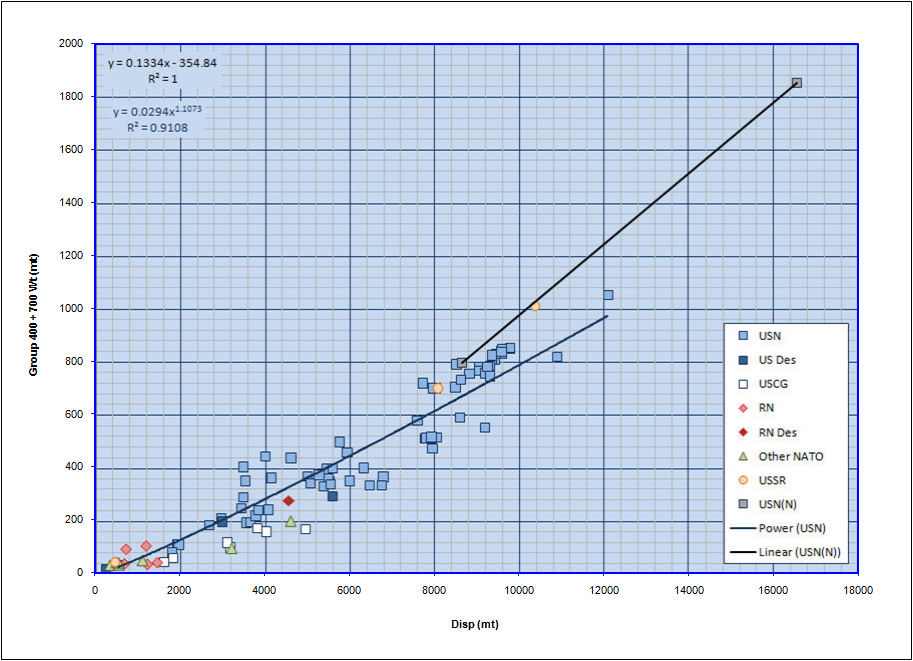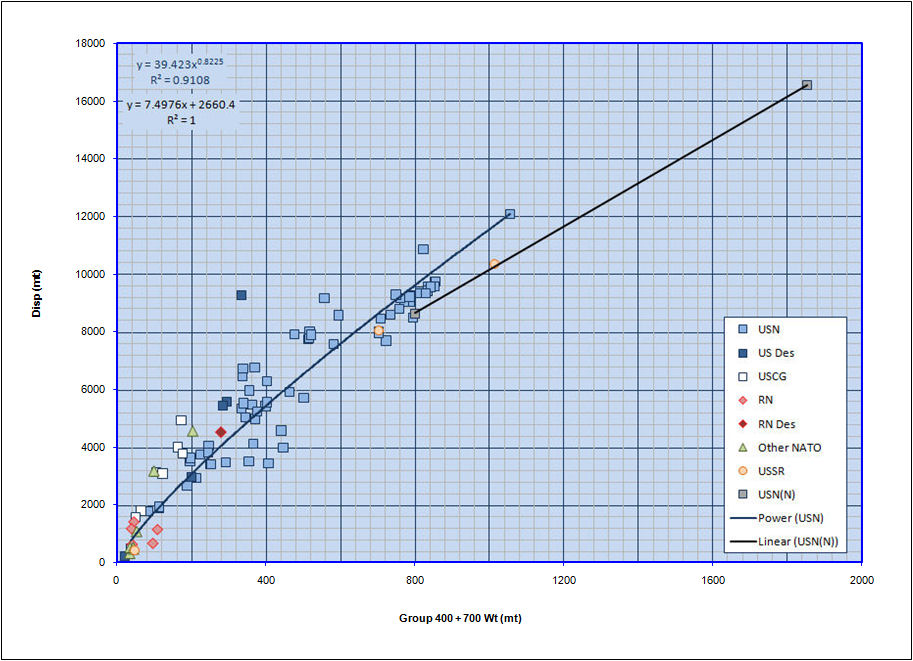Next, here is a figure relating vessel length (on waterline) to displacement.  It can be used to estimate a design's length based on the overall total displacement of the vessel.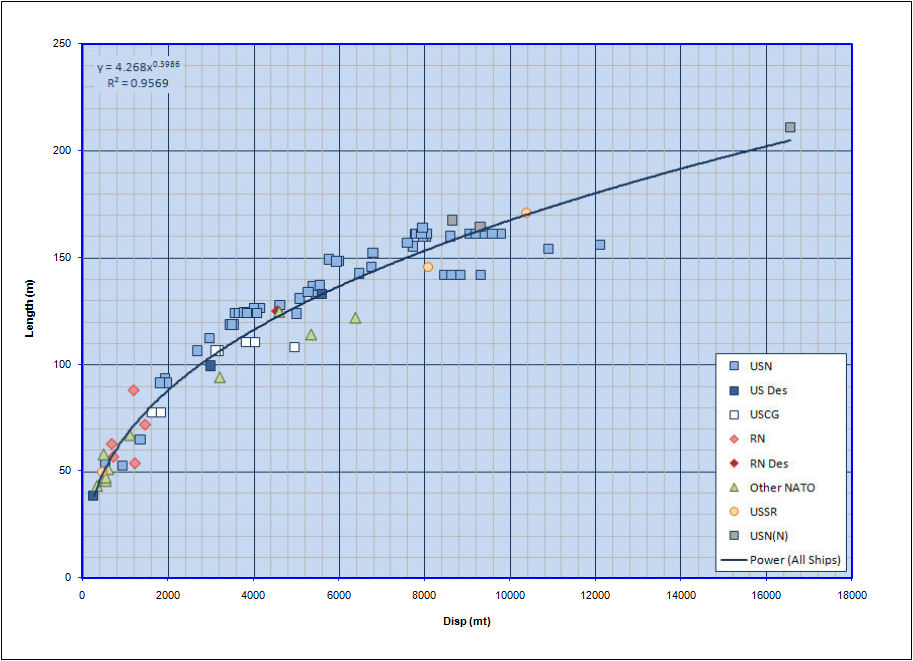Here is a graph of Beam vs Length, which can be used to estimate the beam of a design.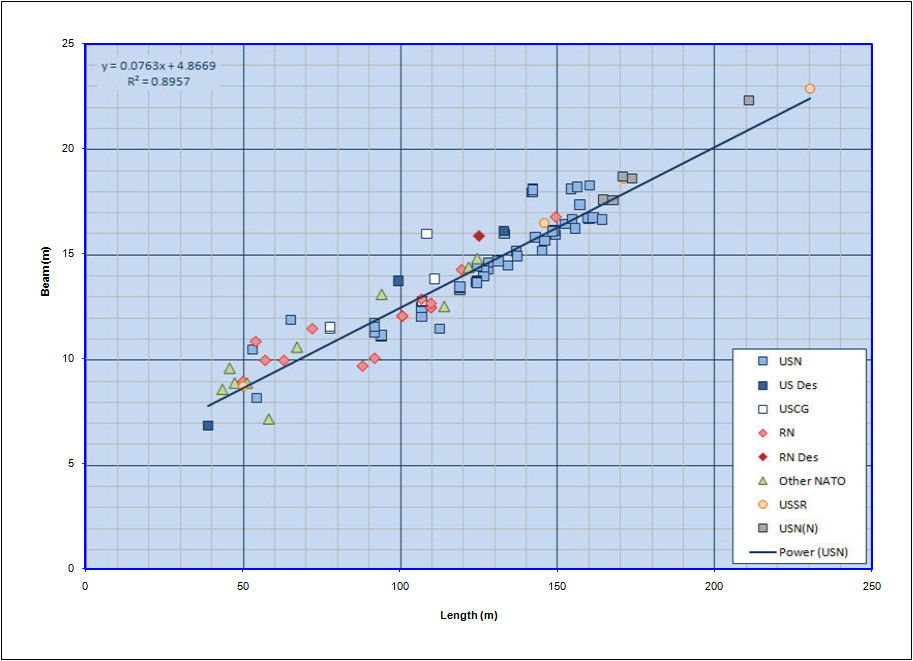Here is a plot of Depth vs Length, which can be used to estimate the depth of a vessels hull (from the keel to the weatherdeck) if that data is not known.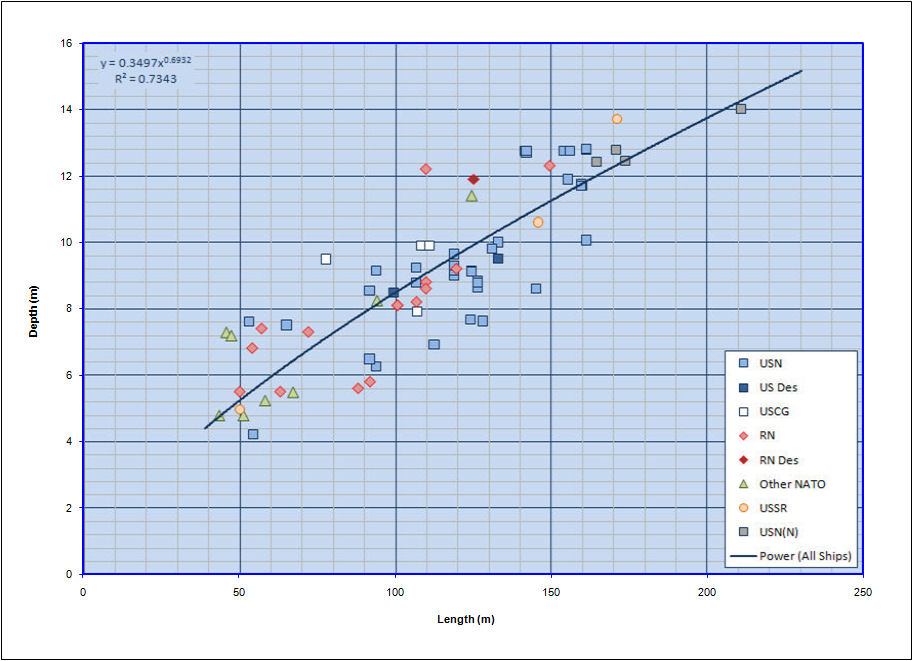Here is a figure relating installed Propulsion Power per Ton Displacement x Speed to a non-dimensional speed for the vessel.  It can be used to make a first estimate of the total power that will be required for the vessel, based on its total displacement and intended maximum service speed.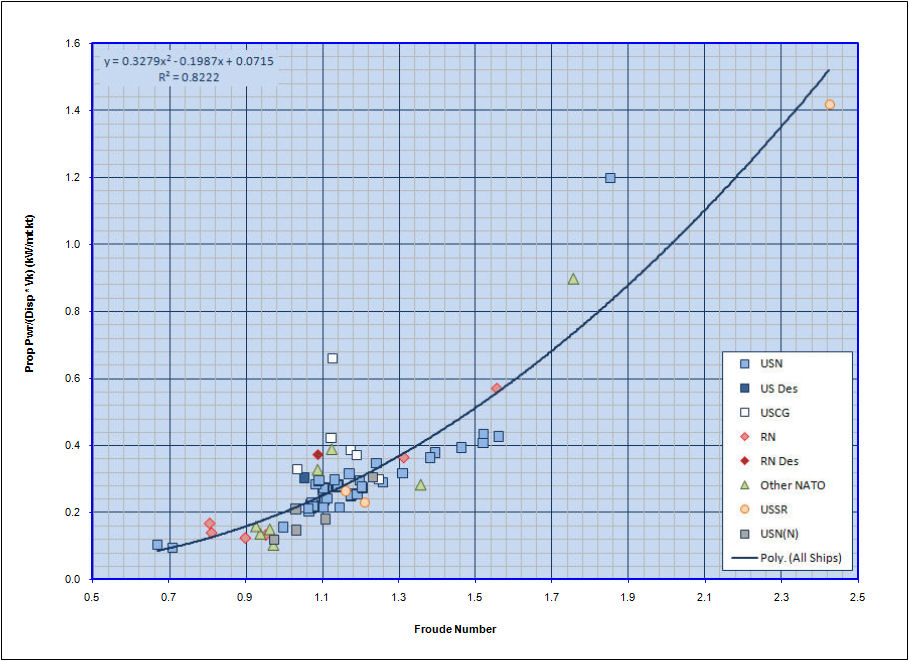Additionally, I have also included a second figure directly relating installed power to a "powering coefficient" as a secondary (backup) means of making an initial estimate of required installed power.  Here the "powering coefficient" is defined as:

Pcoef = 1 / ( Vk ^ 3 * Disp ^ (2/3))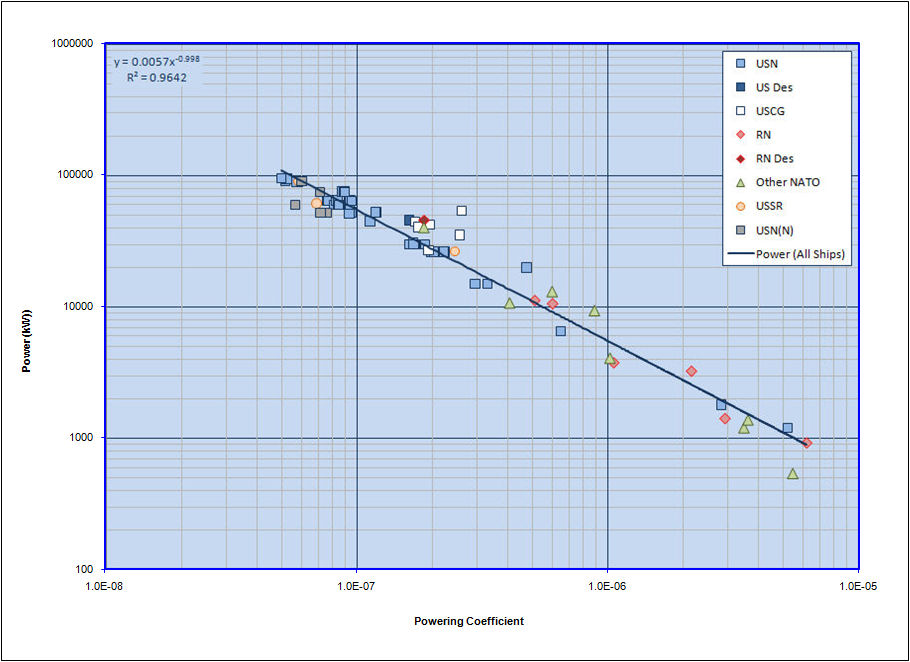Here is a figure relating total installed Generating Power versus Full Load Displacement.  It can be used to make an initial rough estimate of the total installed generating power that will be required onboard a vessel.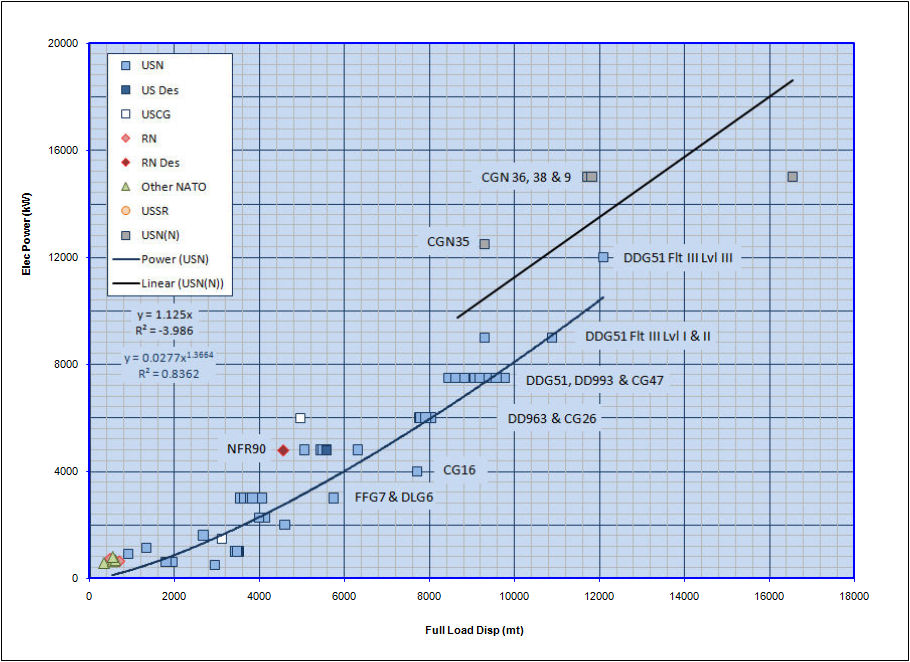And finally, here is a figure relating the total number of accommodations on a vessel divided by the total displacement of the vessel (# accommodations/mt) versus the year the vessel entered service (or was intended to enter service for design studies).  It can be used to make a first estimate of total crew accommodations onboard a vessel.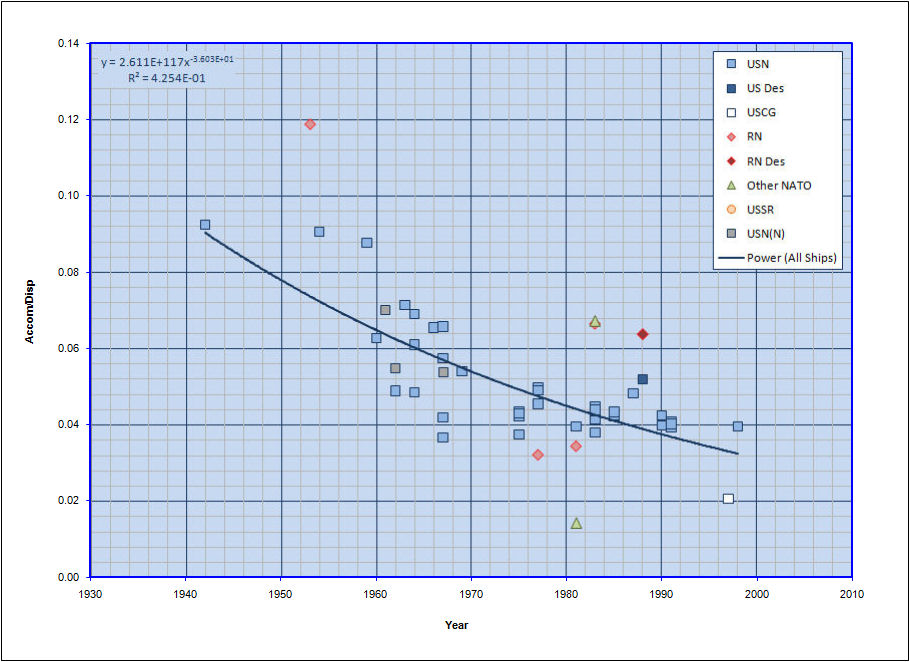Home